# 目的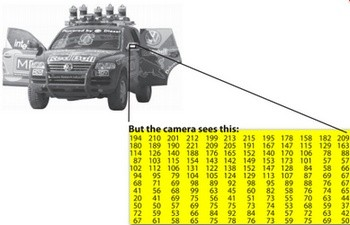# Mat

Mat A, C;                                 // 只创建信息头部分
Mat B(A);                                 // 使用拷贝构造函数
C = A;                                    // 赋值运算符


Mat D (A, Rect(10, 10, 100, 100) ); // using a rectangle
Mat E = A(Range:all(), Range(1,3)); // using row and column boundaries


Mat F = A.clone();
Mat G;
A.copyTo(G);


 OpenCV函数中输出图像的内存分配是自动完成的（如果不特别指定的话）。
使用OpenCV的C++接口时不需要考虑内存释放问题。



# 存储方式

RGB是最常见的，这是因为人眼采用相似的工作机制，它也被显示设备所采用。
HSV和HLS把颜色分解成色调、饱和度和亮度/明度。这是描述颜色更自然的方式，比如可以通过抛弃最

YCrCb在JPEG图像格式中广泛使用。
CIE Lab*是一种在感知上均匀的颜色空间，它适合用来度量两个颜色之间的 距离 。

# 显式地创建一个 Mat 对象

Mat 不但是一个很赞的图像容器类，它同时也是一个通用的矩阵类，所以可以用来创建和操作多维矩

## Mat() 构造函数

    Mat M(2,2, CV_8UC3, Scalar(0,0,255));
cout << "M = " << endl << " " << M << endl << endl;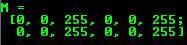CV_[The number of bits per item][Signed or Unsigned][Type Prefix]C[The channel number]

    int sz = {2,2,2};
Mat L(3,sz, CV_8UC(1), Scalar::all(0));


IplImage* img = cvLoadImage("greatwave.png", 1);
Mat mtx(img); // convert IplImage* -> Mat


Create() function: 函数

    M.create(4,4, CV_8UC(2));
cout << "M = "<< endl << " "  << M << endl << endl;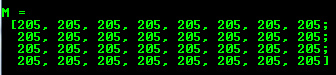MATLAB形式的初始化方式： zeros(), ones(), :eyes() 。使用以下方式指定尺寸和数据类型：

    Mat E = Mat::eye(4, 4, CV_64F);
cout << "E = " << endl << " " << E << endl << endl;

Mat O = Mat::ones(2, 2, CV_32F);
cout << "O = " << endl << " " << O << endl << endl;

Mat Z = Mat::zeros(3,3, CV_8UC1);
cout << "Z = " << endl << " " << Z << endl << endl;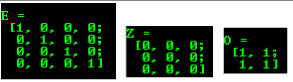Mat C = (Mat_<double>(3,3) << 0, -1, 0, -1, 5, -1, 0, -1, 0);
cout << "C = " << endl << " " << C << endl << endl;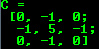Mat RowClone = C.row(1).clone();
cout << "RowClone = " << endl << " " << RowClone << endl << endl;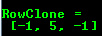# 格式化打印

Note 调用函数 randu() 来对一个矩阵使用随机数填充，需要指定随机数的上界和下界：
Mat R = Mat(3, 2, CV_8UC3); randu(R, Scalar::all(0), Scalar::all(255));

### 默认方式

cout << "R (default) = " << endl <<        R           << endl << endl;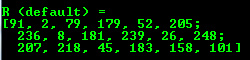### Python

cout << "R (python)  = " << endl << format(R,"python") << endl << endl;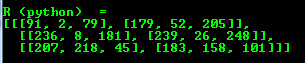### 以逗号分隔的数值 (CSV)

cout << "R (csv)     = " << endl << format(R,"csv"   ) << endl << endl;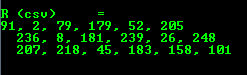### Numpy

cout << "R (numpy)   = " << endl << format(R,"numpy" ) << endl << endl;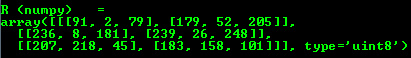### C语言

cout << "R (c)       = " << endl << format(R,"C"     ) << endl << endl;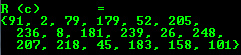# 打印其它常用项目

OpenCV支持使用运算符<<来打印其它常用OpenCV数据结构。

### 2维点

    Point2f P(5, 1);
cout << "Point (2D) = " << P << endl << endl;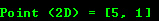### 3维点

    Point3f P3f(2, 6, 7);
cout << "Point (3D) = " << P3f << endl << endl;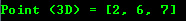### 基于cv::Mat的std::vector

    vector<float> v;
v.push_back( (float)CV_PI);   v.push_back(2);    v.push_back(3.01f);
cout << "Vector of floats via Mat = " << Mat(v) << endl << endl;### std::vector点

    vector<Point2f> vPoints(20);
for (size_t E = 0; E < vPoints.size(); ++E)
vPoints[E] = Point2f((float)(E * 5), (float)(E % 7))
cout << "A vector of 2D Points = " << vPoints << endl << endl;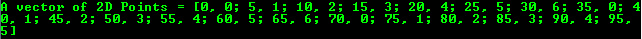©️2019 CSDN 皮肤主题: 创作都市 设计师: CSDN官方博客